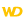WINDEV, WEBDEV AND WINDEV MOBILEONLINE HELPVersion: 15161718192021222324
 Home |Sign in | EnglishHelpWLanguageWLanguage functionsControls, pages and windowsDrawing functions (Image controls and variables)Object syntaxFunctions for drawing on Image variables (object syntax)DrawRectangleGradientPresentationExampleGradient parametersRelated examplesSee alsoAlphaBlendBackgroundChangeModeCopyCropDominantImageColorDrawArcDrawBorderDrawChordDrawCircleDrawHaloDrawLineDrawPointDrawPolygonDrawPolylineDrawRectangleDrawRectangleGradientDrawRoundedRectangleDrawShadowDrawSliceDrawTextDrawTextRTFEncrustColorEndDrawingFillFontHorizontalSymmetryInvertColorModifyHSLModifyHueModifyLightnessModifySaturationPenPixelColorPixelOpacityResizeRotationSaveBMPSaveGIFSaveJPEGSavePNGStartDrawingVerticalSymmetryNew WINDEV, WEBDEV, WINDEV Mobile 24 feature!
WINDEVWEBDEVWINDEV MobileOthersIn french: <Variable Image>.DessineRectangleDégradé
Draws a rectangle in an Image variable.
Example
MyImage is Image = "Test.gif"
// Draw a rectangle whose background changes from red to blue
// via yellow
MyImage.DrawRectangleGradient(10, 20, 100, 150, LightRed, LightBlue, 0, LightYellow, 30)
IMG_MyDrawing = MyImage

Syntax

Drawing a rectangle by specifying the points of the rectangle and the gradient

<Image variable>.DrawRectangleGradient(<X1> , <Y1> , <X2> , <Y2> , <Start color> , <End color> [, <Angle> [, <Color 3> [, <Distance color 3> [, <Color 4> [, <Distance color 4>]]]]])
<Image variable>: Image variable
Name of Image variable to use.
<X1>: Integer
X coordinate (in pixels) of top left corner of rectangle.
<Y1>: Integer
Y coordinate (in pixels) of top left corner of rectangle.
<X2>: Integer
X coordinate (in pixels) of bottom right corner of rectangle.
<Y2>: Integer
Y coordinate (in pixels) of bottom right corner of rectangle.
<Start color>: Integer or constant
Start color of gradient. This color corresponds to:
<End color>: Integer or constant
End color of gradient. This color corresponds to:
<Angle>: Optional integer
Angle that will be used for the selection of colors in the gradient. The angle value is included between 0 (horizontal angle, default value) and 360°.
<Color 3>: Optional constant or integer
Color that will be used in the gradient. This color corresponds to:
<Distance color 3>: Optional integer
Percentage separating the start color from color 3.
<Color 4>: Optional constant or integer
Color that will be used in the gradient. This color corresponds to:
<Distance color 4>: Optional integer
Percentage separating the start color from color 4.Drawing a rectangle by specifying the points of the rectangle and by using the Background type

<Image variable>.DrawRectangleGradient(<X1> , <Y1> , <X2> , <Y2> , <Gradient>)
<Image variable>: Image variable
Name of Image variable to use.
<X1>: Integer
X coordinate (in pixels) of top left corner of rectangle.
<Y1>: Integer
Y coordinate (in pixels) of top left corner of rectangle.
<X2>: Integer
X coordinate (in pixels) of bottom right corner of rectangle.
<Y2>: Integer
Y coordinate (in pixels) of bottom right corner of rectangle.
Name of the Background variable that contains the characteristics of the gradient.Drawing a rectangle by using the Rectangle type and by specifying the gradient

<Image variable>.DrawRectangleGradient(<Rectangle> , <Start color> , <End color> [, <Angle> [, <Color 3> [, <Distance color 3> [, <Color 4> [, <Distance color 4>]]]]])
<Image variable>: Image variable
Name of the Image variable to use.
<Rectangle>: Rectangle variable
Name of Rectangle variable that defines the coordinates of the rectangle to draw.
<Start color>: Integer or constant
Start color of gradient. This color corresponds to:
<End color>: Integer or constant
End color of gradient. This color corresponds to:
<Angle>: Optional integer
Angle that will be used for the selection of colors in the gradient. The angle value is included between 0 (horizontal angle, default value) and 360°.
<Color 3>: Optional constant or integer
Color that will be used in the gradient. This color corresponds to:
<Distance color 3>: Optional integer
Percentage separating the start color from color 3.
<Color 4>: Optional constant or integer
Color that will be used in the gradient. This color corresponds to:
<Distance color 4>: Optional integer
Percentage separating the start color from color 4.Versions 21 and laterDrawing a rectangle by using the Rectangle type and the Background type

<Image variable>: Image variable
Name of the Image variable to use.
<Rectangle>: Rectangle variable
Name of Rectangle variable that defines the coordinates of the rectangle to draw.
Name of Background variable containing the characteristics of gradient.New in version 21Drawing a rectangle by using the Rectangle type and the Background type

<Image variable>: Image variable
Name of the Image variable to use.
<Rectangle>: Rectangle variable
Name of Rectangle variable that defines the coordinates of the rectangle to draw.
Name of Background variable containing the characteristics of gradient.Drawing a rectangle by using the Rectangle type and the Background type

<Image variable>: Image variable
Name of the Image variable to use.
<Rectangle>: Rectangle variable
Name of Rectangle variable that defines the coordinates of the rectangle to draw.
Name of Background variable containing the characteristics of gradient.
Remarks

The color parameters are used as follows:The angle is used to define the orientation of the gradient.The gradients performed with 3 or 4 colors are only available for an angle set to 0 or 90 degrees.
Note: The gradient colors are not available for some systems (Windows 98 or Me for example) or for TSE 256 colors. In this case, a rectangle whose color corresponds to <Start color> is displayed.
Related Examples:Unit examples (WINDEV): The drawing functions [ + ] Using the main drawing functions of WINDEV to:- Initialize an Image control for drawing- Draw simple shapes- Write a text into a drawing- Change the color in a drawing
Component : wd240pnt.dll
Minimum version required
• Version 24